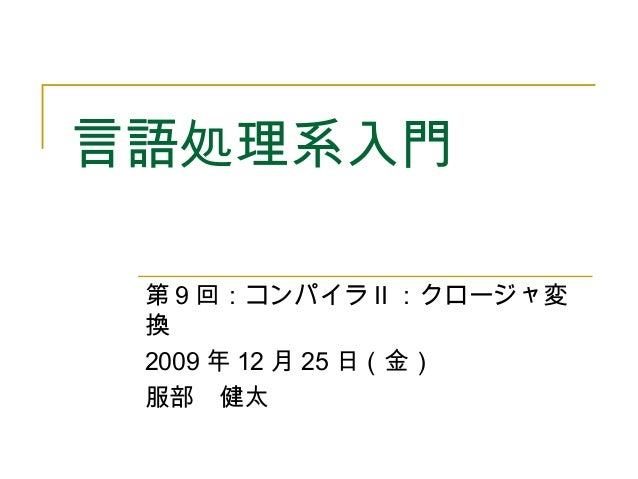Successfully reported this slideshow.Upcoming SlideShare
×

# 言語処理系入門9

591 views

Published on

Published in: Software
• Full Name
Comment goes here.

Are you sure you want to Yes No### 言語処理系入門9

1. 1. 言語処理系入門 第 9 回：コンパイラ II ：クロージャ変 換 2009 年 12 月 25 日（金） 服部　健太
2. 2. クロージャ変換  関数定義から自由変数の参照をなくす  関数は，自由変数が格納されたレコード（クロージャレコー ド）を引数で受け取る．  自由変数の参照はレコードの対応するフィールド参照に置き換 える  例： let sum = (let c = ref 0 in fn n -> c := n) in sum 3 let sum = ( let c = ref 0 in let f clos n = let c = clos in c := n in { f; c } ) in sum sum 3 関数本体に自由変数の参照はない 自由変数 c をクロージャレコードに格納
3. 3. クロージャ表現の例 code v1 : vn code1 v1 : vn code2 code3 v1 : vn code2 vn+1 : vp code1 Vp+1 vq : 1-block closure 2-block closures Linked closures
4. 4. 再帰関数のクロージャ表現の例 code v1 : vn 1-block closure Env.Closure code1 v1 : vn Closure Environment 2-block closure code f v1 : vn code g w1 : wn code f code g v1 : vn w1 : wn let rec f x = … g… and g y = … f …
5. 5. CPS 式の構文（少し変更）  K ::= E  | let x1 = E1 and … and xn = En in K  | let rec x1 = E1 and … and xn = En in K  | if V then K1 else K2  | case V of l1 x1 -> K1 | … | ln xn -> Kn  | V k V | k V  E ::= V  　　 | let x1 = E1 and … and xn = En in E  　　 | let rec x1 = E1 and … and xn = En in E  |{ l1=V1;…; ln = Vn } | l(V)  | fn k x -> K | fn x -> K  | V.l | V1.l <-V2  | op(V1, … ,Vn)  V ::= c | x | k --- ただし， k, x∈Variable 関数呼び出しや分岐を 伴わない単純な式
6. 6. ネストした let/let rec の簡約  クロージャ変換の前に， let x = (let y = E1 in E2) in E3 のようにネストした let 式を let y = E1 in let x = E2 in E3 のような形に変換しておく  このとき， y のスコープが E3 にまで拡大するので，もし ， E3 に y の自由な出現があった場合， y を rename （ α 変換）する必要がある．  let x = (let y = E1 in E2) in E3 ⇒ let y’ = E1 in let x = E2[y←y’] in E3  let rec の場合は以下のように変換する  let rec x = (let y = E1 in E2) in E3 ⇒ let rec y’ = E1 and x = E2[y←y’] in E3
7. 7. クロージャ変換後の式の構文  K ::= E  | let x1 = E1 and … and xn = En in K  | let rec x1 = E1 and … and xn = En in K  | if V then K1 else K2  | case V of l1 -> K1 | … | ln -> Kn  | p V k V | p k V  E ::= V  |{V1;…; Vn }  | code p k x = K in E  | V#l | V1#l <- V2  | op(V1, … ,Vn)  V ::= c | x | k --- ただし， k, x∈Variable  l ∈ Nat
8. 8. クロージャ変換  関数定義 [[fn x1 … xn -> K]] ⇒ code f clos x1 … xn = let y1 = clos#1 and … ym = clos#m in [[K]] in { f; y1; … ; ym }  関数適用 [[V V1 … Vn]] ⇒ let f = V#0 in f V V1 … Vn y1, y2, … ym は関数定 義に含まれる自由変数
9. 9. 自由変数の定義  FV(E) は式 E に出現する自由変数の集合であ り，以下のように再帰的に定義される  FV(c) = φ  FV(x) = {x}  FV(fn x -> E) = FV(E)/{x}  FV(let x = E1 in E2) = FV(E1) FV(∪ E2)/{x}  FV(let rec x = E1 in E2) = (FV(E1) FV(∪ E2))/{x}  FV(E1 E2) = FV(E1) FV(∪ E2)
10. 10. レコードの変換  フィールドラベル付きのレコードをラベルなしのレ コードに変換する  ラベルのかわりに位置でフィールドにアクセスする  クロージャ変換ではないが，ついでにやってしまう  事前にラベルと位置の対応表を作っておく．  型検査のフェーズなど  レコード生成  [[l1=V1;…; ln = Vn ]] ⇒{V1;…; Vn }  フィールド参照  [[V.l]] ⇒ V#pos_of(l)  [[V1.l<-V2]] ⇒ V1#pos_of(l)<-V2
11. 11. タグ付きデータの変換  タグ付きデータをレコードに変換する  タグに対応する整数値とデータの２つのフィールドを含んだレ コードで表現  例： @Foo(3) ⇒ { 0; 3 }  事前にタグにあらかじめ整数値を割当てておく  こちらも型検査フェーズなどで  タグ付きデータ生成  [[l(V)]] { num_of(l); V }⇒  case 式  [[case V of l1 x1 -> K1 | … | ln xn -> Kn]] ⇒ let x = V#0 in case x of num_of(l1) -> let x1 = V#1 in [[K1]] | …
12. 12. いくつかの最適化  再帰呼び出しの場合，クロージャから関数のコード ポインタをいちいち取り出す必要はない．  code fact’ fact n =  … let fact’ = fact#0 in fact’ fact (n-1) …  code fact’ fact n =  … fact’ fact (n-1) …  呼び出し先の関数がわかっていて（直接関数を呼び 出す場合），かつその関数が自由変数を含まない場 合，クロージャを渡す必要はない  let f x = x + x in f (f 10)  自由変数を含まないで，かつ， escape しない関数 はクロージャ生成は不要
13. 13. 参考： lambda lifting  クロージャを作る代わりに，自由変数を引数で明示的に渡すよ うに変換する．  例： let rec sum n = if n = 1 then 1 else let f x = n + x in 　　　　　　　 f (sum (n - 1)) in sum 100 ⇒ let rec sum n = if n = 1 then 1 else let f w x = w + x in 　　　　　　　 f n (sum (n - 1)) in sum 100 n は自由変数 自由変数を受け 取る引数を追加
14. 14. 演習問題  今週のサンプルプログラムを動かしてみよ  講義で紹介した最適化を実装せよ
15. 15. 次回予定  日時：  2010 年 1 月 8 日（金） 10 ： 30 － 12 ： 00  場所：  LB2 3F/C1  内容：  コンパイル III ：コード生成，実行時ライブラリ ， GC